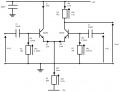# Differential amplifer frequency responce prediction

#### Mitchy190

Joined Apr 23, 2012
33
Hey! I was wondering, how would I predict the frequency response of the circuit below when v2 is grounded?

I have actually measured the response which is also shown below, v2 is grounded.

I know the input impedance of the circuit and therefore I calculated the frequency response of the capacitor and input impedance, which form an RC circuit.

This gives the same shape as my measured response which i would expect, but there is an offset, as you can see. I calculated a 'gain' to be around 27-28 which if i were to multiply the transfer function i worked by, the correct offset would occur.

I have no idea what this is, it cannot not be the Gdm or Gcm of the transistor as i have calculated them and not got 27-28?

I got:

Gdm = R4/2*rE = 164, where rE = 25mV/IE = 25mV/1mA

Gcm = R4/2*R3 = 0.603

Please could someone check this out?Schematic diagram of circuit, v2 is grounded:Graphs:
View attachment frequency responses.pdf

#### #12

Joined Nov 30, 2010
18,190
I'm not the expert in this field, but I don't see any capacitors in your math. Frequency limits in this kind of circuit are always about capacitance. Do you know what Miller effect is?

•Mitchy190

#### Mitchy190

Joined Apr 23, 2012
33
I calculated the frequency response by working out the transfer function of the capacitor and the input impedance.

This attachment to this reply shows what I mean:

View attachment Figure one shows the input impedance of the circuit.pdf

So i have taken into account the capacitor, but to answer your question i have not her of that effect? Could this be taken into account for this situation?

#### Mitchy190

Joined Apr 23, 2012
33
And thank you for your reply!#### #12

Joined Nov 30, 2010
18,190

Edit: Wrong answer. I thought you were talking about the high end.

Last edited:

#### Mitchy190

Joined Apr 23, 2012
33
Okay cheersI will check that out.

#### Audioguru

Joined Dec 20, 2007
11,251
Frequency response involves a low frequency cutoff and a high frequency cutoff.
YOU DID NOT SAY WHICH ONE but later you are talking about the low frequency cutoff which is caused by the value of the input coupling capacitor, the value of R1 parallel to the value of the input impedance of the left transistor and both resistances are in series with the resistance of the signal source.

#### Mitchy190

Joined Apr 23, 2012
33
Yes I mean the low frequency cut off, which occurs around 100Hz. 1/RC.
Okay, I don't fully understand what you mean? I know R1 is in parallel with the input impedance.. do you mean when we represent the circuit as its thevenin equivalent, the voltage source is in series with R thevenin (which is input impedance)?

#### #12

Joined Nov 30, 2010
18,190
If you're talking about the low frequency end, my answers are very wrong.

#### Mitchy190

Joined Apr 23, 2012
33
You see the graph I have put up, one is a graph I measured, by grounding V2, then by varying the frequency and measuring the input and output amplitudes.Do you know how I would predict this outcome? Or an outcome for this situation? This would be extremely helpful! My attempt at this was to look at the low cut off, but that is wrong, i just can see from this result that the graphs have the same shape.. but the one i measured is shifted up by an amount or a gain? I calculated this to be around 28, and wondered if this number could help me predict the outcome i want.

#### Audioguru

Joined Dec 20, 2007
11,251
I don't fully understand what you mean? I know R1 is in parallel with the input impedance.. do you mean when we represent the circuit as its thevenin equivalent, the voltage source is in series with R thevenin (which is input impedance)?
The resistance of the signal source is in series with R1 and the input impedance of the left transistor in parallel.

If your signal source is from an opamp then the series resistance is almost zero.